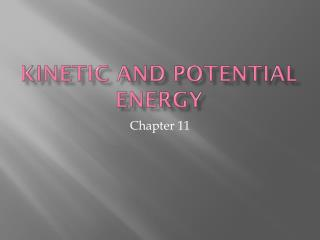DownloadDownload PresentationKinetic and Potential Energy

# Kinetic and Potential Energy

Download Presentation## Kinetic and Potential Energy

- - - - - - - - - - - - - - - - - - - - - - - - - - - E N D - - - - - - - - - - - - - - - - - - - - - - - - - - -
##### Presentation Transcript

1. Kinetic and Potential Energy Chapter 11

2. Definitions: • Kinetic Energy – energy of motion • Formula: KE = 1/2mv2 • Units of KE =the joule • m = mass in kg • v = velocity in m/s

3. Potential Energy – energy of position, or stored energy • Formula: Ug = mgh • Units of Ug are the joule • m = mass in kg • g = gravity (9.8 m/s2) • h = height in meters

4. Other things… • With potential energy, the reference level is important. Remember, you may not always start off at 0 meters. • Velocity is what affects KE the most. Why? Look at the formula… • Height is what determines potential energy. Should the mass or gravity of an object change?

5. Other things continued… • An object has the most KE when it is moving the (fastest/slowest)? • Ug will be greatest when an object is (highest/lowest) and (moving/not moving)? • Energy is conserved. This means energy is converted from KE to Ug and vice-versa.

6. Example 1 • A 90 kg rock climber climbs 45 m up to the top of a hill. He then walks 85 m from the top of the hill to the bottom. If the initial height is the reference level, find the Ug at the top of the hill and at the bottom.

7. Here we go again… Reference level start finish

8. At the top: • m = 90 kg • g= 9.8 m/s2 • h = 45 m • Ug = mgh • Ug = 90 x 9.8 x 45 • Ug = 39,690 J

9. At the bottom: • m= 90 kg • g= 9.8 m/s2 • h= 40 m ???? • Ug = mgh • Ug = 90 x -9.8 x 40 • Ug = -35,280 J

10. Example 2 • A 50 kg artillery shell is shot from a cannon at Earth’s surface to a height of 425 m. • What is the gravitational potential energy of the system when the shell is at this height? • What is the change in potential energy when it falls to a height of 225 m?

11. Answers… • a. 208,250 J • b. -98,000 J

12. Where is Kinetic energy the greatest? The least?

13. Classwork p. 269 #’s 38- 40, 42, 46-49 • Converting km/hr to m/s : multiply by 1000 then divide by 3600? • Use F = ma to find mass • Quiz Thursday!